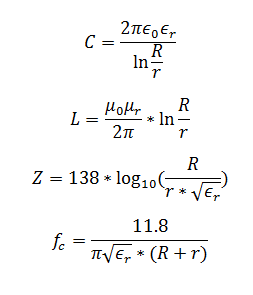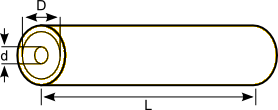# Coaxial Cable Calculator

This CalcTown calculator calculates various parameters of a co-axial cable

#### Result

F
H
Ω
HzWhere

μ0 = 4*3.14159265359 x 10-7

μr = Relative Permeability of the medium

0 = 8.856 x 10-12

r = Relative Permittivity of the medium

R = Outer radius of wire

r = Inner radius of wire

C = capacitance per unit length

L = inductance per unit length

Z = impedance per unit length

fc = Cut-off frequency

*NOTE - Consider μr = 1 for non-magnetic medium, which may not be the case always.